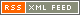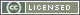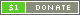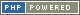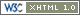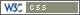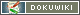# Array

An Array in PTPScript is a collection of items. Each item has a key, and a value. The value is accessed by specifying the key. Keys can be Numbers or Strings, but values can be any PTPScript type, including another Array. However, it should be noted that all Keys of type Float are truncated to Integers, and Booleans used as keys will be converted to Integers.

Arrays are a very important data type, as most of the information made available to the template by the backend programmer will probably be stored in Array trees.

### Creation

Arrays are created using square bracket syntax.

For example:

`{ a = [] }`

The above example simply creates an empty array. In order to specify some contents, each item of the array needs to be separated by a comma.

For example:

`{ a = [5, 9, 14, "hello", 2, foo, true] }`

The above example would create an array with the following values:

• `5` (a Number)
• `9` (a Number)
• `14` (a Number)
• `"hello"` (a String)
• `2` (a Number)
• `foo` (a Variable - can be any value type)
• `true` (a Boolean)

The number of items in an Array can be found by using the `count()` function.

The brackets can be nested, so we can create Arrays within Arrays:

`{ a = [5, 9, 14, "hello", 2, [27, 9], foo, true] }`

#### Keys

Array keys can be specified by using the colon key assignment operator - `:`.

For example:

`{ a = [1: 3, 5: 6, 19: 4, "key": "value"] }`

The above example would create an array with the keys specified. If keys are not specified, then integers are used, starting at 0 and incrementing by 1 (or continuing from the last numerical key used). But, not only do keys not have to be specified, values also do not have to be given.

For example:

`{ a = [4: 5, 9, 10:, "greeting": "hello", 2, [27, 9], foo:, true] }`

If a key is given, and not a value, the key is automatically set to Boolean `false`.

Repeated commas with no key or value between them are simply ignored.

### Access

Assuming that an Array has been assigned to a Variable, its contents can be accessed by using either square bracket syntax or dot syntax. If an Array has been created "temporarily", it cannot be accessed unless it is assigned at some point.

For example:

`{ a = [5, 9, 14] }`

The array created in the example above has been assigned to Variable `a`. Because no keys were specified, the automatically-assigned keys will be 0, 1, and 2, so we can access the values using those keys.

For example:

`{ a }`

The above example would display the contents of the item in Array `a` that has a key of `1`, which would give a value of `9`. We can also use dot syntax for this, even though the key is a number.

For example:

`{ a.1 }`

The above example would acheive the same result as the previous example using square brackets.

One key difference is that Strings and Variables are handled differently depending on the access method used. For more information, see the Square Brackets versus Dots section below.

### Modification

Array contents can be changed using the same methods used to access them, just like any Variable.

For example:

```{ a = [5, 9, 14] }
{ a = 12 }```

The above example would modify the value of the item with key `2`. The same can be acheived by using dot syntax.

If no key is given, a new item is added to the Array.

For example:

```{ a = [5, 9, 3] }
{ a[] = 4 }```

The above example would assign the Number `4` to the next key available for Array `a`, which would be `3`. As with all areas of PTPScript syntax, whitespace does not matter. (Whitespace only matters to separate things that would otherwise get confused, like when saying `a and b`.)

### Square Brackets versus Dots

Dot notation is very useful, as it is very easy to read and often a nice time-saver. However, there are some cases where square brackets have to be used, because dots cannot handle everything. One reason is that dots can only work with keys that are "statically" Strings or Numbers - whereas square brackets can work on "dynamic" keys that are the value of Variables, or the result of expressions. That is because square brackets get treated just like parentheses, except the result is used as an Array key.

For example:

```{ a = [5, "key": "val"] }
{ a.0 }
{ a.key }```

The above example works fine with dot syntax, because we only want to access items whose keys we know. Anything after the dot is treated as a Number or a String - without quotes. Quotes are not only not required, they are explicitly forbidden here. Because of that, keys to be used with dot notation cannot contain whitespace. Square brackets are the solution in such situations.

For example:

```{ a = [5, "key": "val", "another key": "another value"] }
{ b = "key" }
{ a[5 - 5] }
{ a[b] }
{ a["another key"]```

Notice from the example above that just like anywhere else in PTPScript, Variables such as `b` are different to Strings. By putting `b`, we use the value held by Variable `b`. To use a String, we need to explicitly state so by enclosing the string sequence with quotes. Dot notation is the only situation where strings are not surrounded by quotes. So saying `a[b]` is different to `a.b` - the latter is the same as `a["b"]`.

Also in the above example, note that expressions can be used to determine that key that is looked for.

### Mixing Dots and Brackets

Dots can be mixed with square brackets for convenience.

For example:

`{ a.b.c[4 * 5].d }`

Be careful of the syntax!

### Converting to Array

To explicitly convert a value to Array, the Array typecast operator - `@` - should be used. Some operators, such as the Array merge operator - `@` - also explictly convert a value to an Array. (Yes, they are the same symbol, but the former is a prefix operator, and the latter is an infix operator. See Operators for more information.)

When converting to Array, the value will become an item in a new Array (with a key of 0), unless it is already an Array, in which case it will remain unchanged. Therefore typecasting to an Array can be used to ensure that the value is an Array.